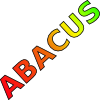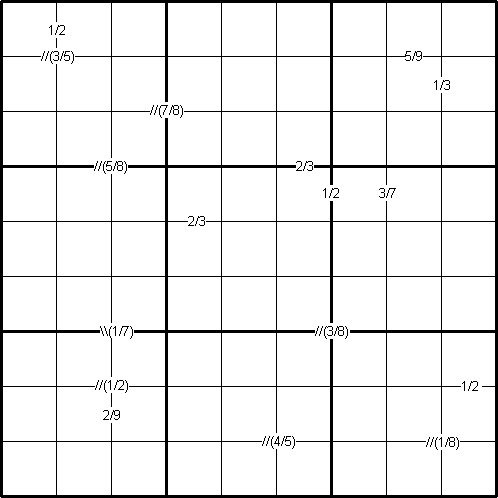#### You may also like### Consecutive Numbers

An investigation involving adding and subtracting sets of consecutive numbers. Lots to find out, lots to explore.### Tea Cups

Place the 16 different combinations of cup/saucer in this 4 by 4 arrangement so that no row or column contains more than one cup or saucer of the same colour.### Counting on Letters

The letters of the word ABACUS have been arranged in the shape of a triangle. How many different ways can you find to read the word ABACUS from this triangular pattern?

# Ratio Sudoku 3

##### Age 11 to 16 Challenge Level:
By Henry Kwok#### Rules of Ratio Sudoku

Like the standard sudoku, this sudoku variant has two basic rules:
1. Each column, each row and each box (3x3 subgrid) must have the numbers 1 to 9.
2. No column, row or box can have two squares with the same number.
The puzzle can be solved with the help of small clue-numbers which are either placed on the border lines between selected pairs of adjacent squares of the grid or placed after slash marks on the intersections of border lines between two diagonally adjacent squares.

The small clue-numbers are ratios or fractions of two digits in the two squares that are horizontally or vertically or diagonally adjacent to each other.

Each fraction is written in its lowest terms, with the smaller number denoted as the numerator. Thus 1/2 can stand for the following combinations of numbers in the two adjacent cells: 1 and 2, 2 and 1, 2 and 4, 4 and 2, 3 and 6, 6 and 3, 4 and 8 or 8 and 4.
Suppose the answers in the two adjacent cells of a puzzle are actually 7 and 5, the clue-number will be written in the form 5/7 instead of 7/5, otherwise this would give away the answer and the puzzle would be too easy to solve!
The position of each pair of diagonally adjacent squares is indicated by either two forward slash marks // or two backward slash marks \\. For example, the //(4/5) on intersection of border lines between the diagonally adjacent squares (8, 6) and (9, 5) means that possible pairs of numbers in the squares are 4 and 5 or 5 and 4. The \\(1/7) on intersection of border lines between the diagonally adjacent squares (6, 2) and (7, 3) means that possible pairs of numbers in the squares are 1 and 7 or 7 and 1.

The bracket symbol ( ) is used to separate the slash marks // or \\ from the fractions on the border lines between the diagonally adjacent squares. For example, it is obvious that //(3/5) is preferable to //3/5.

Finally, the clue-number 2/3 on the border line betweeen the squares (3, 6) and (4, 6) means that possible pairs of numbers for these squares can be from the following combinations: 2 and 3 or 3 and 2, 4 and 6 or 6 and 4, 6 and 9 or 9 and 6.